### Factors Affecting the Performance of Rectifier Type Instruments

The following are the factors that affect the performance of a tool when used in AC.

• Effects of Waveform - Adjustment of instrument measurement can be made in relation to the RMS voltage and current level. Half wave form feature and full wave adjustment tool adjustment on limited scale. And when a waveform of other form elements works on the device, a waveform error occurs in the reading.

• Effect of Temperature Change - Resistance to the adjustment factor varies with temperature changes. And this repair part property causes an error in the tools.

• Effect of Rectifying Instrument - The repair tool has a limited power supply. It allows high-frequency current to pass through.

• Decreases in Sensitivity - The sensitivity of the AC operating type tool is lower than that of DC performance.

• This Instrument can measure the electrical signal of very low frequencies on radio frequencies.

• The instrument also has the ability to measure electrical signals up to a few mega Hz.

• The sensitivity of the tool is much higher than that of any other type of measuring instrument in A.C. In fact, in order to obtain the highest sensitivity to A.C values, we use corrective type tools.

Applications of Rectifying Instrument

• The following are the applications of the rectifying instrument.

• The device uses a voltage gauge whose width is between 50 - 250 V.

• Used as a milli-ammeter or micro-ammeter.

• Use of the correction tool in the communication circuit to rate.Thus, the loading effect of the ac rectifier voltmeter is higher than that of the dc voltmeter.

## Induction Type Meters

• The voltmeter sensitivity of the adjustment type is less than that of the DC voltmeter sensitivity.

• The principle of operation and construction of induction type meters is very simple and easy to understand which is why these are widely used in measuring energy in the domestic and industrial world. For every entry meter we have two drums produced by two different alternating waves on a metal disk. Due to fluctuations of fluxes there is an emf produced, the locally produced emf (as shown in the picture below) interacts with the alternating current currents leading to the production of torque.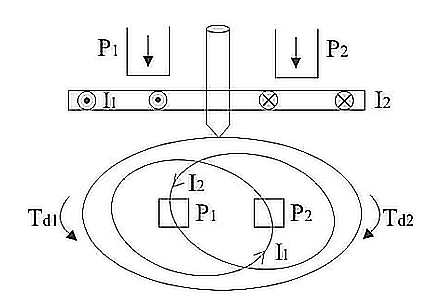• Similarly, the emf produced at the point two interacts with the alternating current at point one, resulting in the production of torque again but in opposite direction. Hence due to these two torques which are in different directions, the metallic disc moves.This is basic principle of working of an induction type meters. Now let us derive the mathematical expression for deflecting torque. Let us take flux produced at point one be equal to F1 and the flux and at point two be equal to F2. Now the instantaneous values of these two flux can written as:

Where, Fm1 and Fm2 are respectively the maximum values of fluxes F1 and F2, B is phase difference between two fluxes. We can also write the expression for induced emf's at point one be

• at point two. Thus we have the expression for eddy currents at point one is

• Where, K is some constant and f is frequency. Let us draw phasor diagram clearly showing F1, F2, E1, E2, I1 and I2. From phasor diagram, it clear that I1 and I2 are respectively lagging behind E1 and E2 by angle A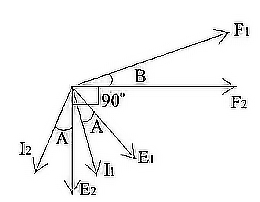• The angle between F1 and F2 is B. From the phasor diagram the angle between F2 and I1 is (90- B+A) and the angle between F1 and I2 is (90 + B + A). Thus we write the expression for deflecting torqueas• The total torque is Td1 - Td2, on substituting the value of Td1 and Td2 and simplifying the expression we get

This is known as the general expression for the deflecting torque in the induction type meters. Now there are two types of induction meters and they are written as follows:

1. Single phase type induction meters.

2. Three phase type induction meters.

Following are the advantages of induction type meters:

• They are inexpensive as compared to moving iron type instruments.

• They have high torque is to weight ratio as compared to other instruments.

• They retain their accuracy over wide range of temperature as well as loads.

• Without proper compensation measures, a considerable amount of errors are caused in the measurement due to temperature, waveform and frequency changes.

• Induction meters can use only for AC measurements.

• They consume a considerable amount of power.

• They have nonlinear scales.

## Extend the range of instruments by use of shunts and Multipliers

There is no fundamental difference in the operating principles of ammeters and voltmeters. Both are current-operated devices (except electrostatic type voltmeters) i.e. deflecting torque is produced when current flows through their operating coils.
In an ammeter, the deflecting torque is produced by the current to be measured or by a definite fraction of it whereas in a voltmeter torque is produced by the current proportional to the voltage to be measured. Thus, the real difference between the two instruments is in the magnitude of the currents producing the deflecting torque. The essential requirements of a measuring instrument are that its introduction into the circuit, where measurements are to be made, does not alter the circuit conditions and the power consumed by them for their operation is small. An ammeter is connected in series with the circuit whose current is to be measured. Therefore, it should have a low resistance. On the other hand, a voltmeter is connected in parallel with the circuit whose voltage is to be measured; therefore, it must have high resistance.

Thus, we conclude that the difference is only in the resistance of the instrument, in fact, an ammeter can be converted into voltmeter by connecting a high resistance in series with it.

It is already seen that the moving coil instruments can carry the maximum current of about 50 mA safely and the potential drop across the moving coil instruments is about 50 mV. However, in practice, heavy currents and voltages are required to be measured. Therefore, it becomes necessary that the current and voltage being measured be reduced and brought within the range of the instrument. There are four common devices used for the range extension of ammeter and voltmeter namely; shunts, multipliers and current and potential transformers. The shunts and multipliers are used to extend the range of moving coil ammeters and voltmeters respectively.

Whereas in the case of moving iron ammeters, for the ranges up to 0 – 250 A, shunts are used and for the ranges higher than that, CTs are used. And also, in the case of moving iron voltmeters, for the ranges up to 0 – 750 V, multipliers are used and for the ranges higher than that, Pts are used.

It is possible to extend the range of an ammeter by using a shunt. A shunt is a low-value resistance having minimum temperature co-efficient and is connected in parallel with the ammeter whose range is to be extended. The combination is connected in series with the circuit whose current is to be measured.This shunt provides a bypath for extra current because it is connected across(i.e. in parallel with) the instrument. These shunted instruments can be used to measure currents many times greater than their normal full-scale deflection currents. The ratio of maximum current (with shunt) to the full-scale deflection current (without shunt) is known as the ‘multiplying power’ or ‘multiplying factor’ of the shunt.

Example: A moving coil ammeter reading up to 1 ampere has a resistance of 0.02 ohm. How could this instrument be adopted to read current up to 100 amperes?
Solution: In this case,

Full-scale deflection current of the ammeter,   Im = 1 A
Line current to be measured,    I  = 100 A
Resistance of ammeter,  Rm = 0.02 ohm
Let, the required shunt resistance = S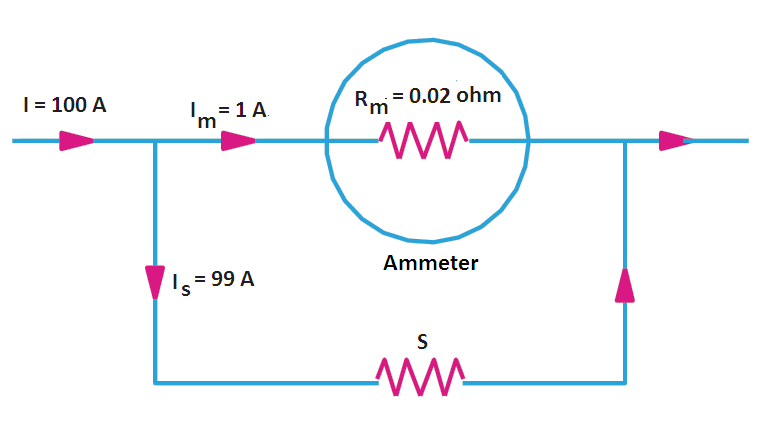As seen from Figure, the voltage across the instrument coil and the shunt resistance is the same since
both are joined in parallel.
∴  Im*Rm = S*Is = S(I − Im)
or  S =  Im*Rm/(I – Im)
= 1*0.02/(100 – 1) = 0.02/99 =  0.000202  Ans.

## Multipliers are used for the range extension of voltmeters. The multiplier is a non-inductive high-value resistance connected in series with the instrument whose range is to be extended. The combination is connected across the circuit whose voltage is to be measured.  Example: A moving coil voltmeter reading up to 20 mV has a resistance of 2 ohms. How this instrument can be adopted to read voltage up to 300 volts.  Solution: In this case,

Voltmeter resistance, Rm = 2 ohm
Full-scale  voltage of the voltmeter, ν = RmIm = 20 mV = 0.02 V
Full-scale deflection current,  Im = v/Rm = 0.02/2 = 0.01 A
Voltage to be measured, V = 300 V
Let the series resistance required = R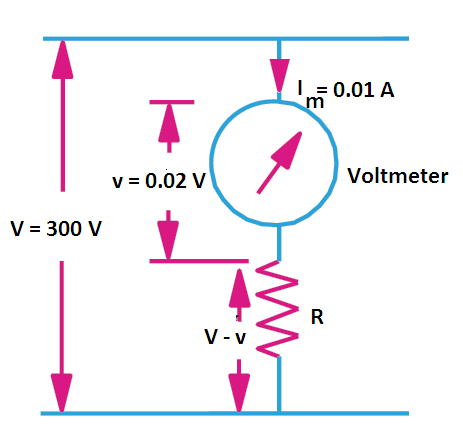Then as seen from figure, the voltage drop across R is V – ν
R *Im = V – ν
or R = (V – v)/Im
or R = (300 – 0.02)/0.01 = 299.98/0.01 = 29998 ohms Ans.

Shunts can not be used to extend the range of moving-iron AC ammeters accurately. It is because the division of current between the operating coil and the shunt varies with frequency (since reactance of the coil depends upon frequency). In practice, the range of moving-iron AC ammeters are extended by one of following methods: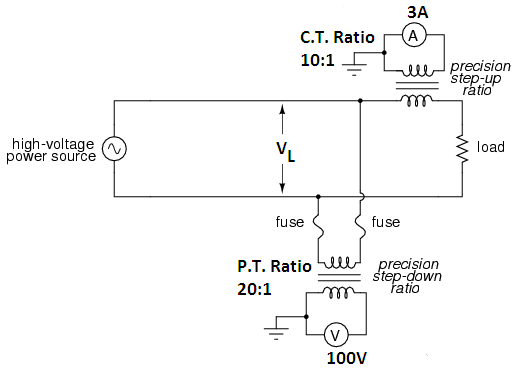1. Range Extension of Ammeter by Coil Turns:
By changing the number of turns of the operating coil. For example, suppose that full-scale deflection is obtained with 400 ampere-turns. For full-scale reading with 100A, the number of turns required would be = 400 / 100 = 4. Similarly, for full-scale reading with 50A, the number of turns required in = 400/50 = 8.
Thus the ammeter can be arranged to have different ranges by merely having a different number of turns on the coil. Since the coil carries the whole of the current to be measured, it has a few turns of thick wire. The usual ranges obtained by this method are from 0 -250 A.

2. Range Extension of Ammeter by Current Transformer:
For ranges above 0 – 250 A, a current transformer is used in conjunction with 0 – 5 A moving iron AC ammeter as shown in the figure. The current transformer is a step up transformer i.e. number of secondary turns is more than the primary turns.Usually, the primary winding of the transformer contains a single turn or at the most a few turns. The primary of this transformer is connected in series with the load and carries the load current. The AC ammeter is connected across the secondary of the transformer.
Since in figure, the current transformer ratio is 10:1, it means that line (or load) current is equal to 10 times the reading on the AC meter. Therefore, load current, IL = 3 x 10 = 30 A.

3. Range Extension of Voltmeter by Potential Transformer:
The range of a moving-iron AC voltmeter is extended by connecting a high resistance (multiplier) in series with it.For ranges higher than 0 – 750 V, where power wasted in the multiplier would be excessive, a 0 – 110 V moving-iron AC voltmeter is used in conjunction with a potential transformer as shown in the figure.The potential transformer is a step-down transformer i.e. number of primary turns is more than the secondary turns. The primary of the transformer is connected across the load across which voltage is to be measured. The AC voltmeter is connected across the secondary.Since in figure, the potential transformer ratio is 20:1, the load voltage is equal to 20 times the reading on the AC voltmeter.
Load voltage, VL = 100 x 20 = 2000 V
Note that both secondaries of the instrument transformers are grounded as a safety measure.